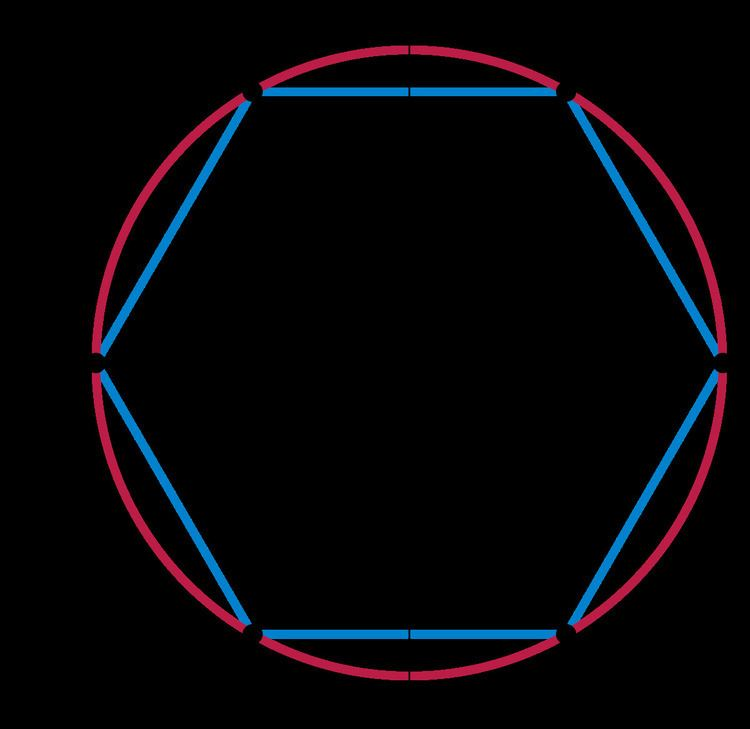# Mathieu group M12

Updated on
Covid-19In the area of modern algebra known as group theory, the Mathieu group M12 is a sporadic simple group of order

## Contents

26 · 33 ·· 11 = 95040 = 12×11×10×9×8 ≈ 1×105.

## History and properties

M12 is one of the 26 sporadic groups and was introduced by Mathieu (1861, 1873). It is a sharply 5-transitive permutation group on 12 objects. Burgoyne & Fong (1968) showed that the Schur multiplier of M12 has order 2 (correcting a mistake in (Burgoyne & Fong 1966) where they incorrectly claimed it has order 1).

The double cover had been implicitly found earlier by Coxeter (1958), who showed that M12 is a subgroup of the projective linear group of dimension 6 over the finite field with 3 elements.

The outer automorphism group has order 2, and the full automorphism group M12.2 is contained in M24 as the stabilizer of a pair of complementary dodecads of 24 points, with outer automorphisms of M12 swapping the two dodecads.

## Representations

Frobenius (1904) calculated the complex character table of M12.

M12 has a strictly 5-transitive permutation representation on 12 points, whose point stabilizer is the Mathieu group M11. Identifying the 12 points with the projective line over the field of 11 elements, M12 is generated by the permutations of PSL2(11) together with the permutation (2,10)(3,4)(5,9)(6,7). This permutation representation preserves a Steiner system S(5,6,12) of 132 special hexads, such that each pentad is contained in exactly 1 special hexad, and the hexads are the supports of the weight 6 codewords of the extended ternary Golay code. In fact M12 has two inequivalent actions on 12 points, exchanged by an outer automorphism; these are analogous to the two inequivalent actions of the symmetric group S6 on 6 points.

The double cover 2.M12 is the automorphism group of the extended ternary Golay code, a dimension 6 length 12 code over the field of order 3 of minimum weight 6. In particular the double cover has an irreducible 6-dimensional representation over the field of 3 elements.

The double cover 2.M12 is the automorphism group of any 12×12 Hadamard matrix.

M12 centralizes an element of order 11 in the monster group, as a result of which it acts naturally on a vertex algebra over the field with 11 elements, given as the Tate cohomology of the monster vertex algebra.

## Maximal subgroups

There are 11 conjugacy classes of maximal subgroups of M12, 6 occurring in automorphic pairs, as follows:

• M11, order 7920, index 12. There are two classes of maximal subgroups, exchanged by an outer automorphism. One is the subgroup fixing a point with orbits of size 1 and 11, while the other acts transitively on 12 points.
• S6:2 = M10.2, the outer automorphism group of the symmetric group S6 of order 1440, index 66. There are two classes of maximal subgroups, exchanged by an outer automorphism. One is imprimitive and transitive, acting with 2 blocks of 6, while the other is the subgroup fixing a pair of points and has orbits of size 2 and 10.
• PSL(2,11), order 660, index 144, doubly transitive on the 12 points
• 32:(2.S4), order 432. There are two classes of maximal subgroups, exchanged by an outer automorphism. One acts with orbits of 3 and 9, and the other is imprimitive on 4 sets of 3.
• Isomorphic to the affine group on the space C3 x C3.
• S5 x 2, order 240, doubly imprimitive on 6 sets of 2 points
• Centralizer of a sextuple transposition
• Q:S4, order 192, orbits of 4 and 8.
• Centralizer of a quadruple transposition
• 42:(2 x S3), order 192, imprimitive on 3 sets of 4
• A4 x S3, order 72, doubly imprimitive, 4 sets of 3 points.
• ## Conjugacy classes

The cycle shape of an element and its conjugate under an outer automorphism are related in the following way: the union of the two cycle shapes is balanced, in other words invariant under changing each n-cycle to an N/n cycle for some integer N.

## References

Similar Topics
Stop, Youre Killing Me
Alan Burnett